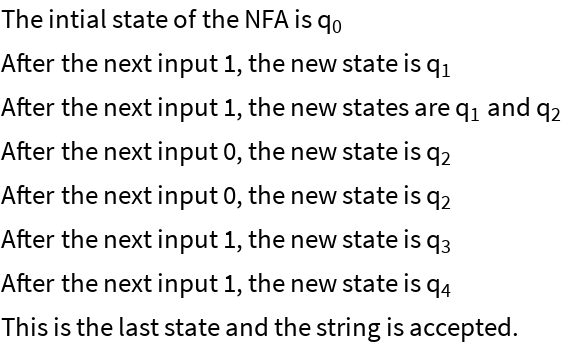#Function Repository Resource:

# NFASimulation

Simulate the behavior of a nondeterministic finite automaton

Contributed by: Katja Della Libera
 ResourceFunction["NFASimulation"][rules,alphabet,start,accept,input] simulates the finite automaton described by rules, alphabet, start and accept over input.

## Details and Options

Rules can be specified similarily to the format in TuringMachine or NFAPlot, as {currentstate, input}{newstate(s)}.
The alphabet, accept states and input should be given as lists.

## Examples

### Basic Examples

A simple NFA that rejects the given input:

 In:=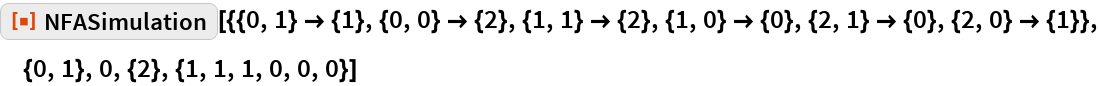Out=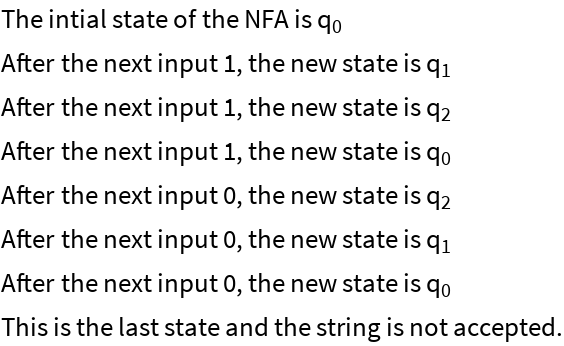If the last state is in the list of accept states, the input is accepted:

 In:=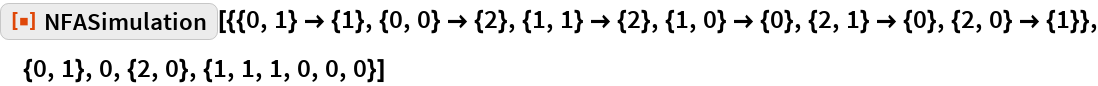Out=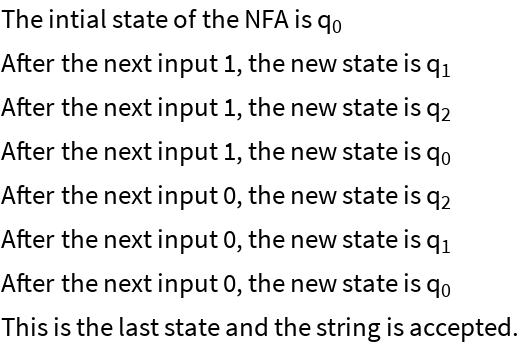If one of the letters in the input is not in the alphabet, the simulation will throw an error:

 In:=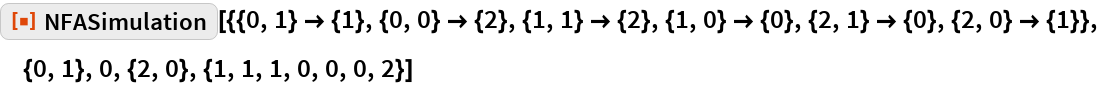Out=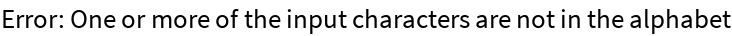### Scope

ResourceFunction["NFAPlot"] can illustrate the NFA before simulation:

 In:=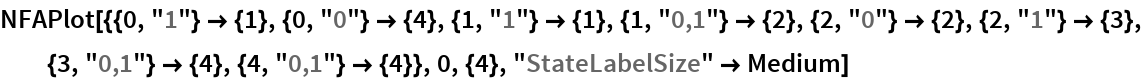Out=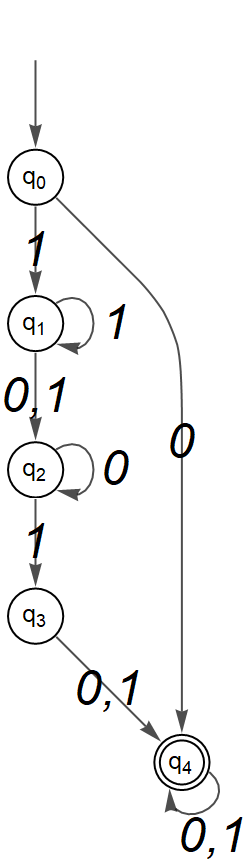In:=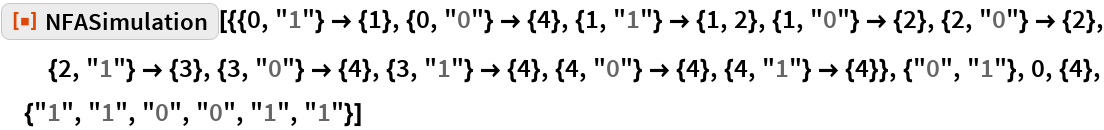Out=